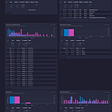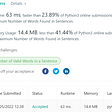# Number of Good Pairs — Day 101(Python)

After a long hiatus, I decided to start writing again as a new year resolution. I wanted to start with something easy, hence choosing the following problem.

## 1512. Number of Good Pairs

Given an array of integers `nums`, return the number of good pairs.

A pair `(i, j)` is called good if `nums[i] == nums[j]` and `i` < `j`.

Example 1:

`Input: nums = [1,2,3,1,1,3]Output: 4Explanation: There are 4 good pairs (0,3), (0,4), (3,4), (2,5) 0-indexed.`

Example 2:

`Input: nums = [1,1,1,1]Output: 6Explanation: Each pair in the array are good.`

Example 3:

`Input: nums = [1,2,3]Output: 0`

Constraints:

• `1 <= nums.length <= 100`
• `1 <= nums[i] <= 100`

This question is very similar to Two Sum. We have solved this problem before. You can find it here.

A brute force method will traverse through the list of numbers and check if the numbers match.

`class Solution:    def numIdenticalPairs(self, nums: List[int]) -> int:        output = 0        for i in range(len(nums)):            for j in range(i+1, len(nums)):                if(nums[i] == nums[j]):                    output += 1        return output`

Complexity

Time Complexity

Since we have 2 for-loops here, hence time complexity is O(N²).

Space Complexity

We are not using any extra space, and hence the space complexity will be O(1).

An optimized method will traverse through the list once, but to save time we use a dictionary to store already traversed elements. The keys will be traversed elements and the values will be the number of times we have seen that number.

`class Solution:    def numIdenticalPairs(self, nums: List[int]) -> int:        output = 0        dict_number = dict()        for n in nums:            if n in dict_number:                output += dict_number[n]                dict_number[n] += 1            else:                dict_number[n] = 1        return output`

Complexity

Time Complexity

Since we have 1 for-loop here. Hence time complexity is O(N).

Space Complexity

We are not using any extra space and hence the space complexity will be O(N).

--

--

--

## More from Annamariya Tharayil

Software Engineer. Find me @ www.linkedin.com/in/annamariya-jt

Love podcasts or audiobooks? Learn on the go with our new app.

## Introduction to Cosmos DB## Why user adoption is so important to company success## Quick Nginx access log dashboard## DISCOVER AGILE MANIFESTO## How to Install macOS 12 Monterey on an Unsupported Mac## Google Cloud Services: All You Need To Know About GCP Services## Are you building a vulnerable tool? — Introduction to shlex module## Annamariya Tharayil

Software Engineer. Find me @ www.linkedin.com/in/annamariya-jt

## Build Array from Permutation — Day 103(Python)## [LeetCode]#2114. Maximum Number of Words Found in Sentences## Must-Have Skills for a Data Scientist## Python Interview Questions for 2022